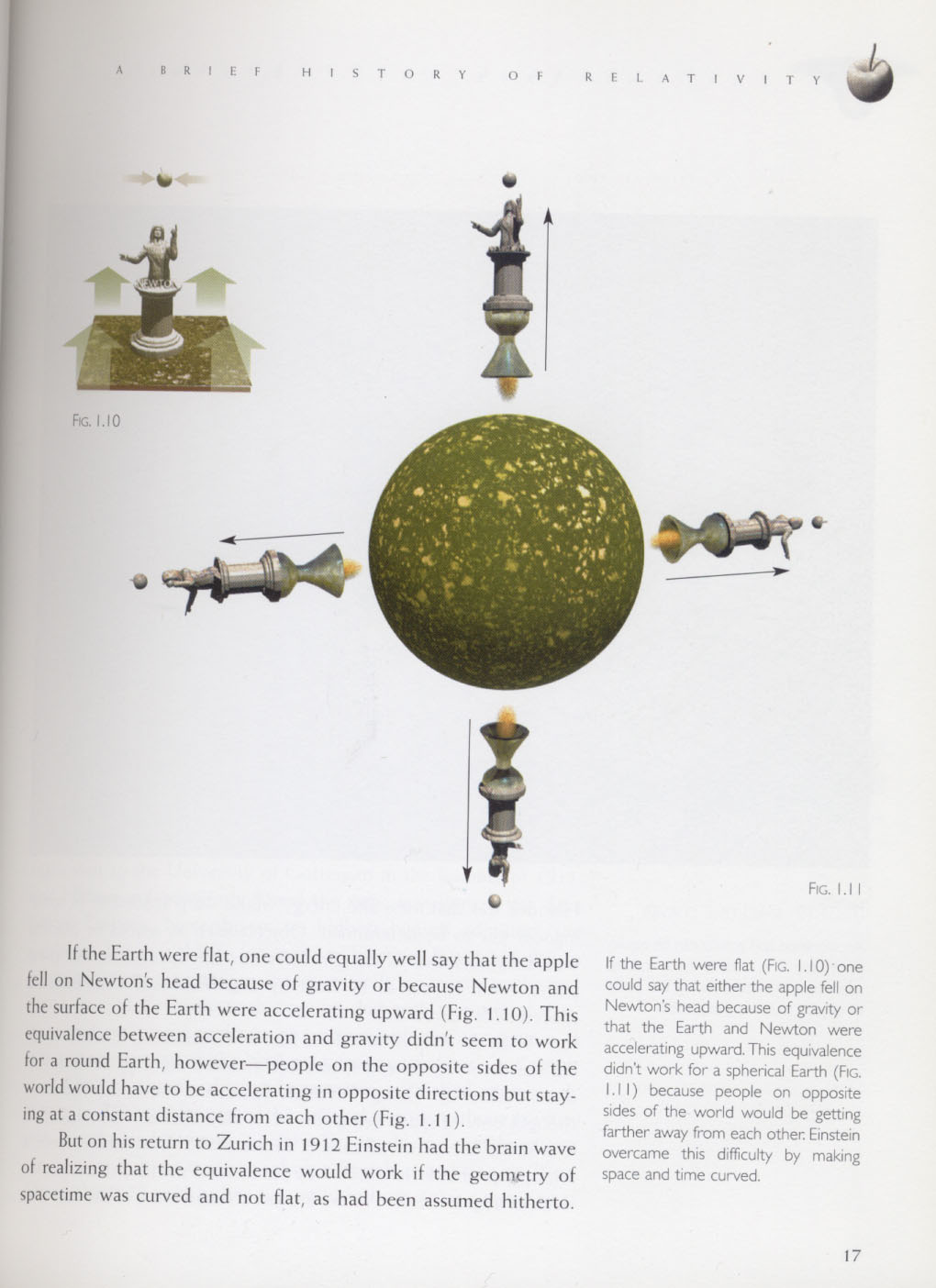Text Size

Jan 29

## Stretched Membrane Black Hole and Cosmic Horizons

Posted by: JackSarfatti
Tagged in: Untagged

Jan 28, 2011, at 8:49 AM, nick herbert wrote:
Two questions
1) where did you get the equation of cosmic acceleration as function of H?
Nick this is textbook general relativity
The covariant acceleration of static LNIFS at fixed "r" in the SSS metrics is
g(r) = (Newtonian "force" acceleration)g00^-1/2
in the case of the de Sitter metric / > 0  in this static representation corresponding to the firing rockets in Hawking's picture (static LNIF detectors)g00 = 1 - / ^2
r = 0 is the observer
i.e. g00 = 0 is the horizon relative to the observer at r = 0.
The Newtonian acceleration is
2c^2/ Therefore the curved spacetime acceleration is
g(r) = 2c^2/ /(1 - / ^2)^1/2
The horizon is at
r = /^-1/2
Let H be a small distance from a horizon from the inside - we are inside our de Sitter horizon
r = /^-1/2 - H
H << /^-1/2
/ = 1/R
then do the algebra, I may have dropped a factor of 2 or so but the basic result is
g(H) ~ c^2/(RH)^1/2
i.e. geometric mean of distance H to the classical horizon surface with square-root of area/entropy of that surface.
2) where does that preposterously small distance L(p) = 10^-33 cm come from?
That comes from Lenny Susskind's "stretched membrane" horizon and basic quantum gravity Heisenberg uncertainty of the metric fluctuation - quantum fuzzy thickness of the classical horizon.
"Three postulates asserting the validity of conventional quantum theory, semi-classical general relativity and the statistical basis for thermodynamics are introduced as a foundation for the study of black hole evolution. We explain how these postulates may be implemented in a “stretched horizon” or membrane description of the black hole, appropriate to a distant observer. The technical analysis is illustrated in the simplified context of 1+1 dimensional dilaton gravity. Our postulates imply that the dissipative properties of the stretched horizon arise from a course graining of microphysical degrees of freedom that the horizon must possess. A principle of black hole complementarity is advocated. The overall viewpoint is similar to that pioneered by ’t Hooft but the detailed implementation is different." http://arxiv.org/pdf/hep-th/9306069v2
Actually Martin may still only need six numbers since the numbers here are derivative as long as he has the Hubble scale as one of the six.
Now one question is what happens to the geodesic observer falling through the black hole? where
g00 = 1 - rs/r
If the observer carries a net surface charge his virtual photons will couple to the virtual electron-positron pairs it seems and he may no longer be a LIF.
We seem to be getting to something like George Chapline's dark star? Maybe nothing can get through the black hole surface and it all smears over the surface as in Lenny Susskind's picture above? Not sure about this.

This does not happen in the subjective cosmological horizon obviously.